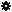﻿ Object Model: NurbsSurface.GetUVFromNormalizedUV2

NurbsSurface.GetUVFromNormalizedUV2

## NurbsSurface.GetUVFromNormalizedUV2### Description

Returns the UV value equivalent to the input normalized UV values. The data is returned in a 1-dimensional array and is ordered the same as for the NurbsSurface.GetUVFromNormalizedUV method output arguments.

Note: This method must be used with scripting languages that don't support arguments passed by reference such as JScript and PerlScript. For more information on getting output arguments, see About Output Argument Arrays.

### C# Syntax

 ```Object NurbsSurface.GetUVFromNormalizedUV2( Double in_dUValueNormalized, Double in_dVValueNormalized ); ```

### Scripting Syntax

 ```oArray = NurbsSurface.GetUVFromNormalizedUV2( UValueNormalized, VValueNormalized ); ```

### Return Value

Array of UV values ordered as UValue, VValue.

### Parameters

Parameter Type Description
UValueNormalized Double The normalized UValue(0.0 to 1.0) from which we want the actual U Value.
VValueNormalized Double The normalized VValue(0.0 to 1.0) from which we want the actual V Value.

### Examples

#### JScript Example

 ```/* JScript example : shows how to retrieve the equivalent of the normalized UV values: .5, .5; from NurbsSurface.GetUVFromNormalizedUV2 */ var oRoot = Application.ActiveProject.ActiveScene.Root; var oSphere = oRoot.AddGeometry( "Sphere", "NurbsSurface" ); // convert VB array to JScript array var vbArgs = new VBArray(oSphere.ActivePrimitive.Geometry.Surfaces(0).GetUVFromNormalizedUV2( .5, .5 )); var args = vbArgs.toArray(); // get the U value LogMessage("U value: " + args ); // get the V value LogMessage("V value: " + args ); ```